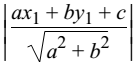# Perpendicular Distance From Point to Straight Line

The length of the perpendicular from the point (x1, y1) to the line ax + by + c = 0 isTo find the length of the perpendicular from the origin, put x1 = 0 and y1 = 0.

The general equation of the straight line is

ax + by + c = 0

y = −(a/b)x − (c/a)

This is of the form y = mx + c

∴ m= − a/b